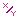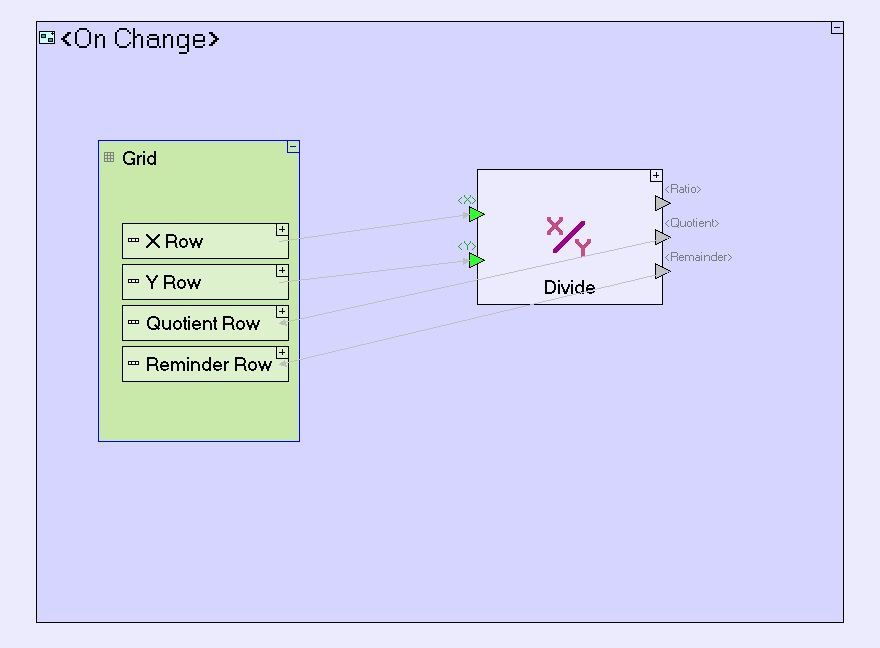Divide - Divide two numbers

This action calculates the ratio between two numbers.

Triggers [both required]

<X> (non-repetitive numeric triggers [required]): The dividend (the number to be divided by <Y>).

<Y> (non-repetitive numeric triggers [required]): The divisor (the number to divide <X> by).

Exits

The default two exits represent two mutually exclusive possibilities:

<Ratio> (non-repetitive numeric exit [required]): The calculated ratio <X> / <Y>.

Division by Zero (error exit [not required]): Charged if <Y> is zero (and thus the ratio cannot be calculated).

To perform integer division, add the following optional exits:

<Quotient> (non-repetitive numeric exit [not required]):the integer value of the division (e.g. if <X> is 13 and <Y> is 5, then <Quotient> will be set to 2).

<Remainder> (non-repetitive numeric exit [not required]):the reminder of the integer division (in the above example of <X>=13 and <Y>=5, <Remainder> will be set to 3).

Usage Examples